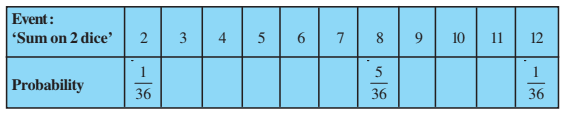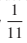Question 22

# Refer to Example 13. (i) Complete the following table:(ii) A student argues that ‘there are 11 possible outcomes 2, 3, 4, 5, 6, 7, 8, 9, 10, 11 and 12. Therefore, each of them has a probabilityDo you agree with this argument? Justify your answer.

(i) Total no. Of outcomes = 36

• (1, 2) and (2, 1) are events for getting a sum as 3

P (E) = 2/36 = 1/18

• (1, 3), (2, 2) and (3, 1) are the events of getting the Sum 4

P(E) = 3/36 = 1/12

• (1, 4), (2, 3), (3, 2) and (4, 1) are the events of getting the sum 5

P(E) = 4/36 = 1/9

• (1, 5), (2, 4), (3, 3), (4, 2) and (5, 1) are the events of Getting a sum 6

P(E) = 5/36

• (1, 6), (2, 5), (3, 4), (4, 3), (5, 2) and (6, 1) are the event of getting a sum 7

P(E) = 6/36 = 1/6

• (3, 6), (4, 5), (5, 4) and (6, 3) are the events of getting a sum 9

P(E) = 4/36 = 1/9

• (4, 6), (5, 5) and (6, 4) are the events of getting a sum 10

P(E) = 3/36 = 1/12

• (5, 6), (6, 5) are the events of getting a sum 11

P(E) = 2/36 =1/18

(ii) No, the eleven sum is not equally likely.